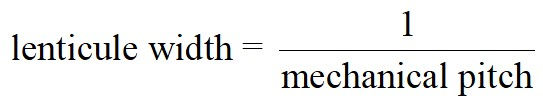top of pageSearch
• Yitzhak Weissman

# Lenticular layouts and the pitches of lenticular sheets

## The lenticular pitch

Every lenticular print expert knows that they must measure the lenticular pitch before creating an interlaced image for print. But what is this pitch?

In my book "Lenticular imaging" I have introduced the concept of the 'optical grid' to explain the origin of the lenticular pitch. The optical grid can be visualized by illuminating a lenticular sheet with a point light source. Such illumination will create thin light lines on the lenticular sheet backplane, one line per lenticule. All lines will be parallel to the lenticules direction. These lines are the optical grid.Figure 1: The optical grid

The lenticular pitch is the density of the optical grid lines, or, in other words, the number of optical grid lines per inch. Figure 1 demonstrates the fact that the lenticular pitch depends on the distance of the point light source from the lenticular sheet.

The lenticular pitch is measured by printing a special pattern and covering it with the lenticular sheet. The lenticular pitch can be determined by viewing the resulting pattern. The value will depend on the viewing distance. This viewing distance corresponds to the distance of the light source of Figure 1 from the sheet.

The lenticular pitch is also called the 'visual pitch'.

## The mechanical pitch

The mechanical pitch is the number of lenticules per inch. It depends only on the properties of the lenticular sheet.

The lenticular pitch must be known for every lenticular print. On the other hand, there is only one (known) application of the mechanical pitch in lenticular print: the creation of layouts.

In a common print layout, images can be positioned arbitrarily. This is not the case in lenticular layouts, because of the centering requirement in lenticular pictures. Since in a layout the centering cannot be adjusted individually for each picture, the images must be positioned in such a manner that centering any one of them will automatically center all. Such layout is called "synchronized". The condition for layout synchronization is that the separation between any two images is a whole multiple of the lenticule width. This condition is illustrated in Figure 2.Figure 2: The condition for layout synchronization

Synchronization may be not essential for a layout of flip images. It is, however, a must for a layout of 3D images.

The lenticule width is simply the inverse of the mechanical pitch:The required precision of the mechanical pitch value for the layout application is the same as of the lenticular pitch. When the point light source of Figure 1 is located at infinity, the optical grid pitch becomes identical to the mechanical pitch, as illustrated in Figure 3.Figure 3: The optical grid for an infinite distance

From Figures 1 and 3 it can be deduced that the mechanical pitch is always greater than the lenticular pitch.

## Mechanical pitch measurement with lenticular pitch measurement methods

One may say that the mechanical pitch can be measured using the known lenticular pitch measurement method with an infinite viewing distance. In practice, a viewing distance that is much greater than the lenticular sheet thickness can 'approximate' infinity. However, the use of a finite distance, regardless of how large it is, will introduce a small error. In some applications, the degree of precision required for the mechanical pitch value may require a viewing distance which is hard to achieve in practice.

A more convenient method could be to use the analytical formula:where t and n are the thickness and the refraction index of the lenticular sheet respectively, V is the viewing distance, and g(V) is the pitch. Using this formula, if the pitch is known for a certain finite viewing distance, it can be computed for infinity, which is the mechanical pitch.

The biggest problem with this method is that it requires the value of the refraction index. This value is not quoted normally by the sheet vendors. If it is unknown, a good guess is 1.58. Another problem is the validity of the analytical formula itself; it is correct only for purely cylindrical designs with the infinity focal plane coinciding with the sheet backplane (see Lenticular Imaging).

## Direct measurements of the mechanical pitch

The lenticule width can be measured directly with a microscope and a linear stage. However, to reach the required precision, a large number of lenticules must be transversed and counted. This requires expensive equipment. The measurement itself is difficult and prone to errors.

In a previous post, I have described how to achieve an infinite viewing distance using a Fresnel lens. This method is shown again in Figure 4 below.Figure 4: Achievement of an infinite viewing distance using a Fresnel lens

An observer located at the focal point of the Fresnel lens will optically appear to the lenticular sheet as being at infinity. This method can be used to measure the mechanical pitch with utmost precision. Figure 5 shows an fPitch pattern for a 20lpi lens. It was photographed from a 50cm distance, and the lenticular pitch is 19.98/inch.Figure 5: Measurement of the lenticular pitch at 50cm

The picture below shows the same arrangement covered by a Fresnel lens and photographed from its focal point. The pitch is now ~20.03/inch. This is the mechanical pitch of this lens sheet.Figure 6: Measurement with a Fresnel lens photographed from the focal distance

The focal length of the Fresnel lens was 1100mm. This is a convenient measurement distance.

In this case, t = 2mm. Choosing n = 1.58, the analytical formula yields a value of 20.03/inch for the mechanical pitch, in perfect agreement with the measurement.

## Summary

A lenticular pitch value is required for every lenticular print. A mechanical pitch value is required for making lenticular print layouts.

Various methods for the measurement of the mechanical pitch are reviewed. A new method based on the use of a Fresnel lens is described.